# Multiplying and dividing by 8

## Home learning focus

In this session you will learn to multiply and divide by 8.

This includes:

• one slideshow
• two activity sheets

# Learn

Click on the slideshow below to solve some pirate problems involving multiplying and dividing by eight.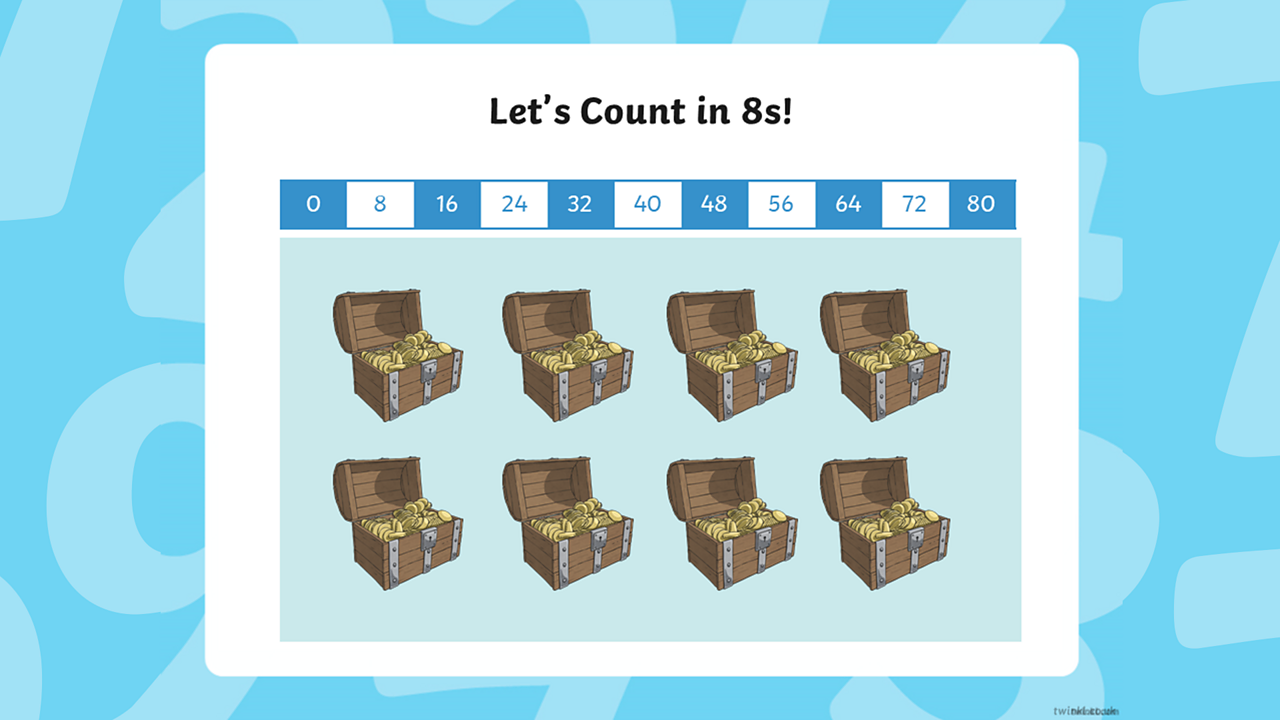Can you count forwards and backwards out loud in eights to 10 × 8 using the counting stick? We can work out unknown multiplication facts by adding or subtracting from known facts. Can you use the counting stick to help work out what 5 × 8 is? If we know 5 × 8, how could this help us to work out 6 × 8? Can you use the counting stick to work out 10 × 8? If we know 10 × 8, how could we work out 9 × 8?1 of 9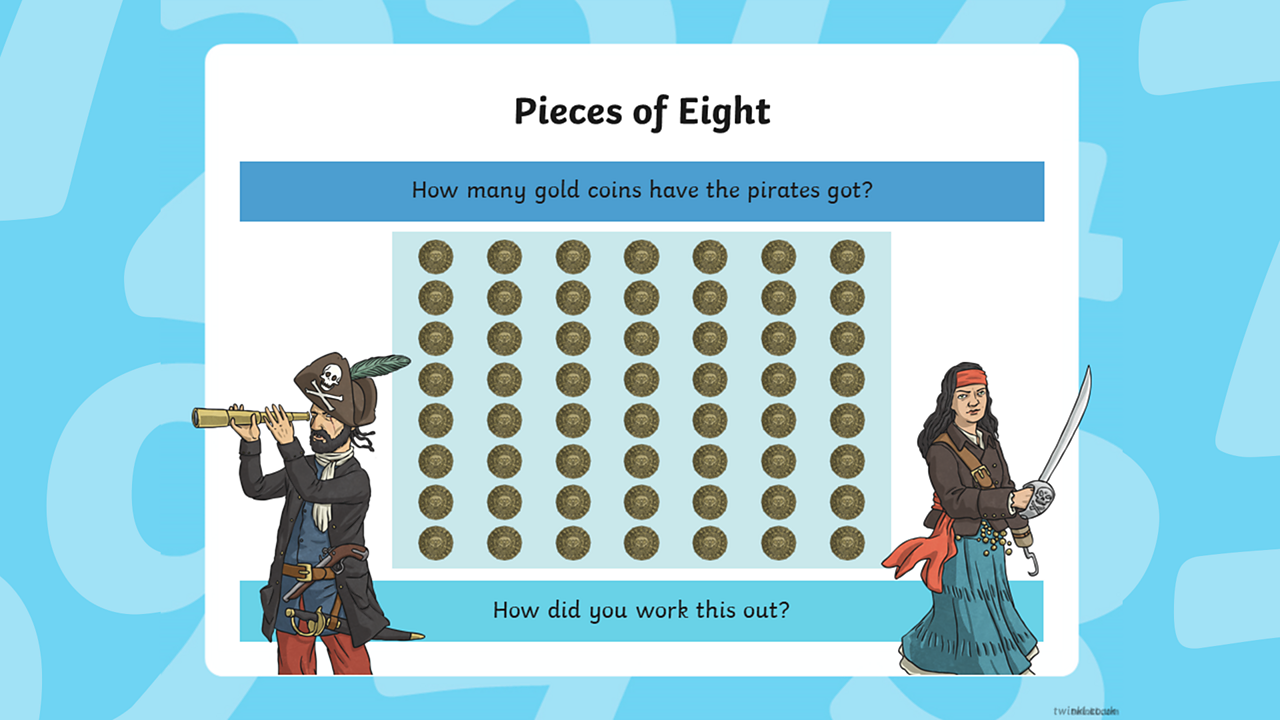How many gold coins have the pirates got? How did you work this out?2 of 9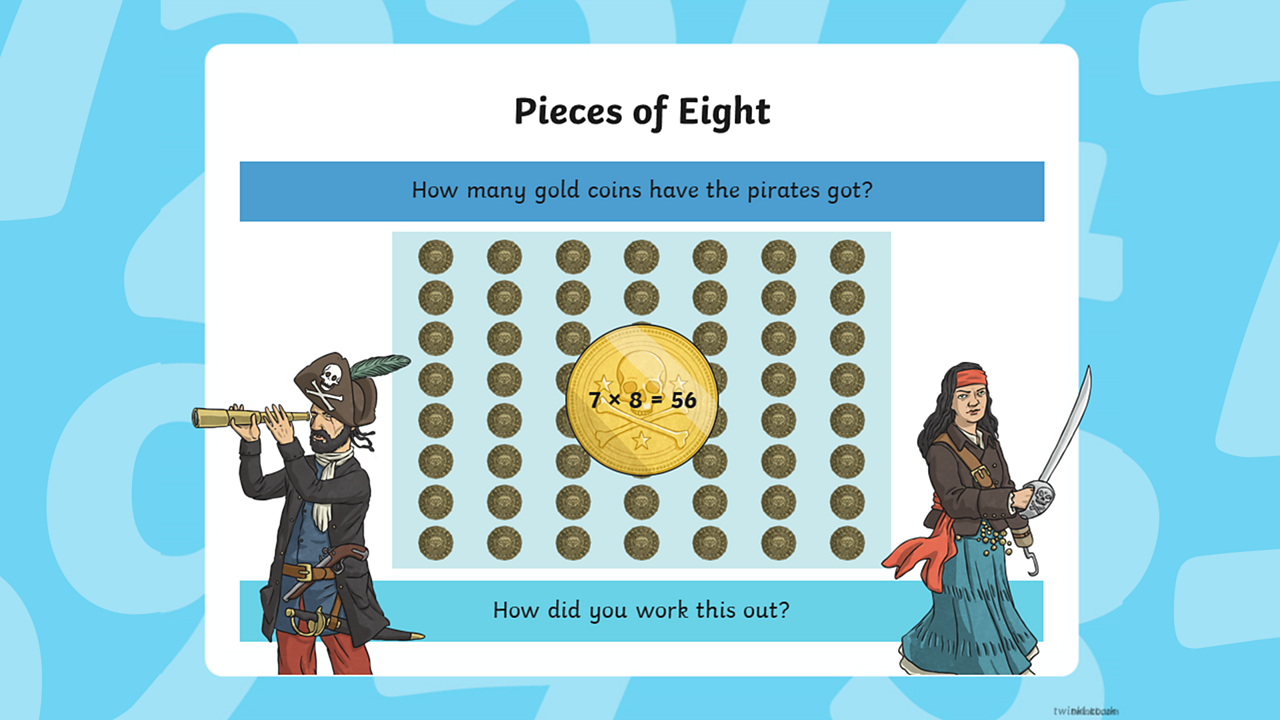The answer is 56. You could write this as 7 × 8 = 56 or it could be written as 8 × 7 = 563 of 9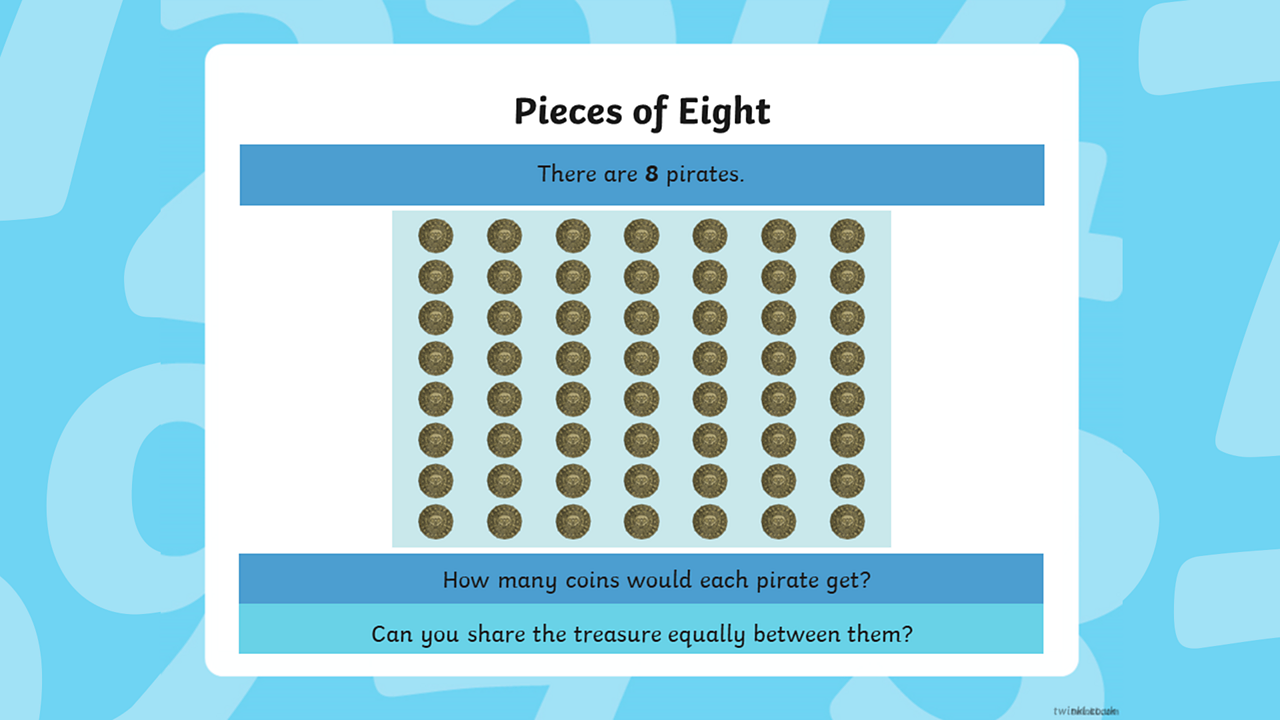There are 8 pirates. How many coins would each pirate get? Can you share the treasure equally between them?4 of 9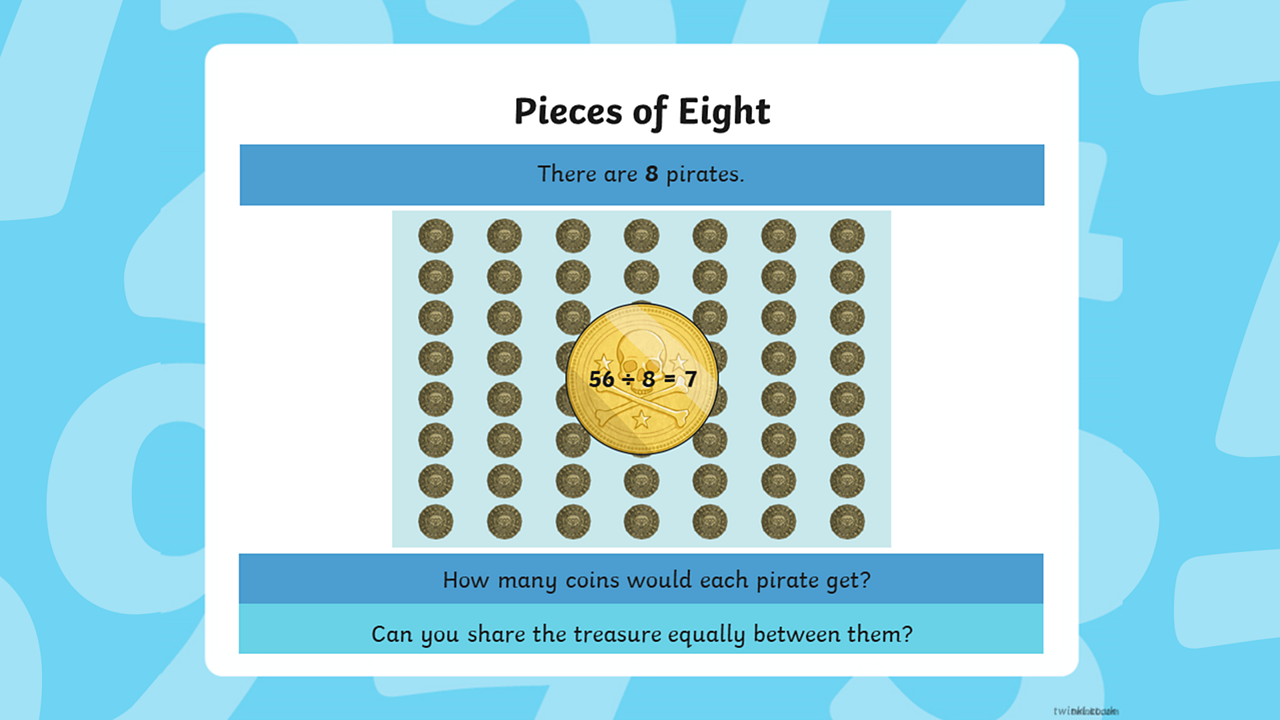The answer is 56 ÷ 8 = 75 of 9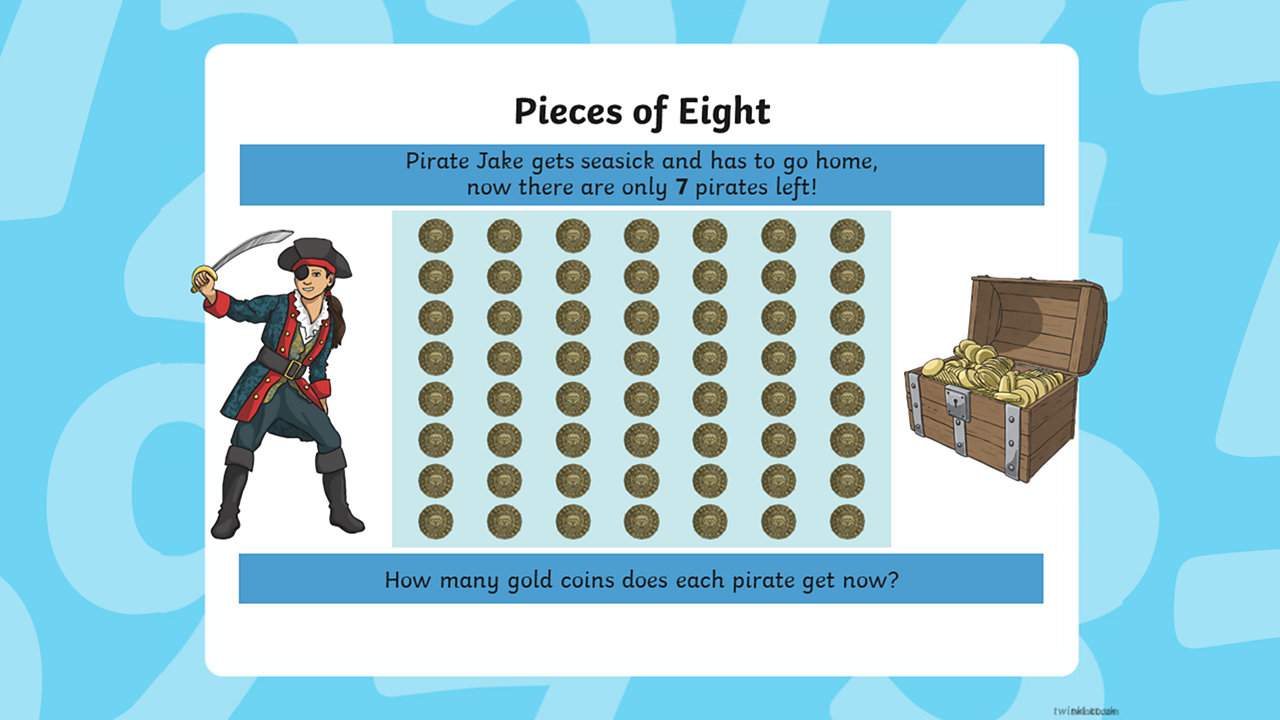Pirate Jake gets seasick and has to go home, now there are only 7 pirates left! How many gold coins does each pirate get?6 of 9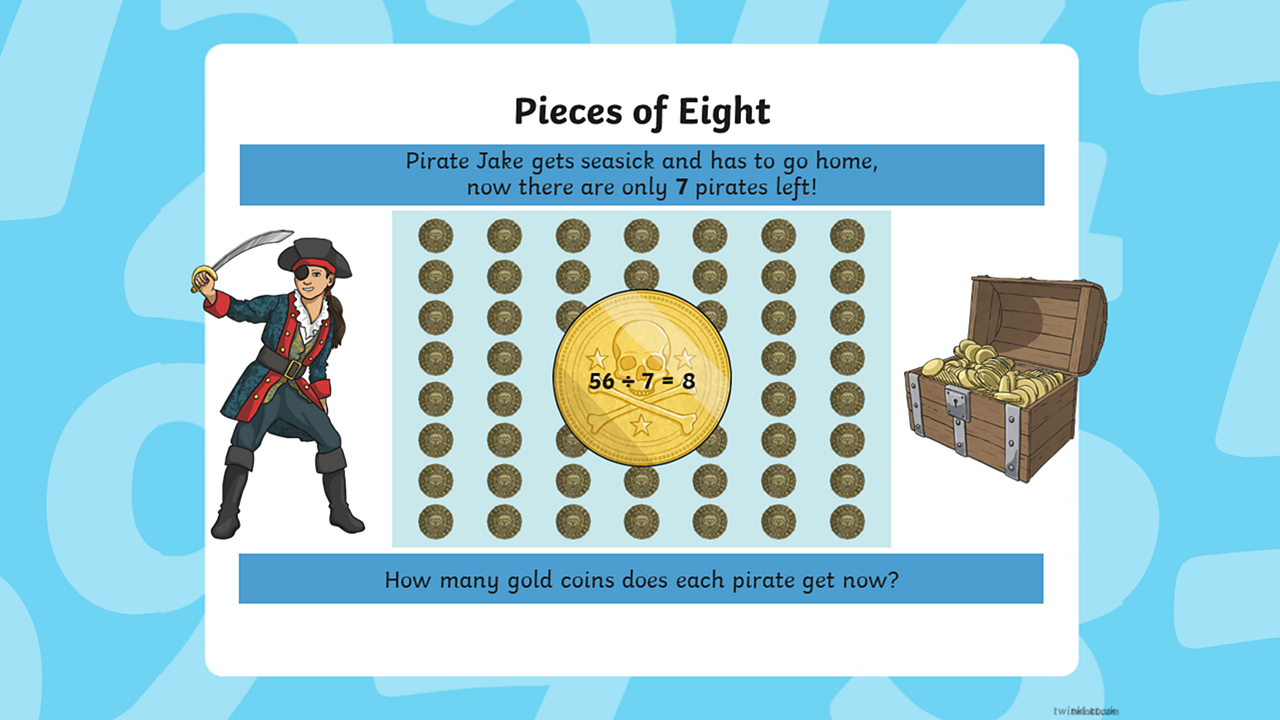The answer is 56 ÷ 7 = 87 of 9What are the multiplication and division facts that describe this array of coins?8 of 9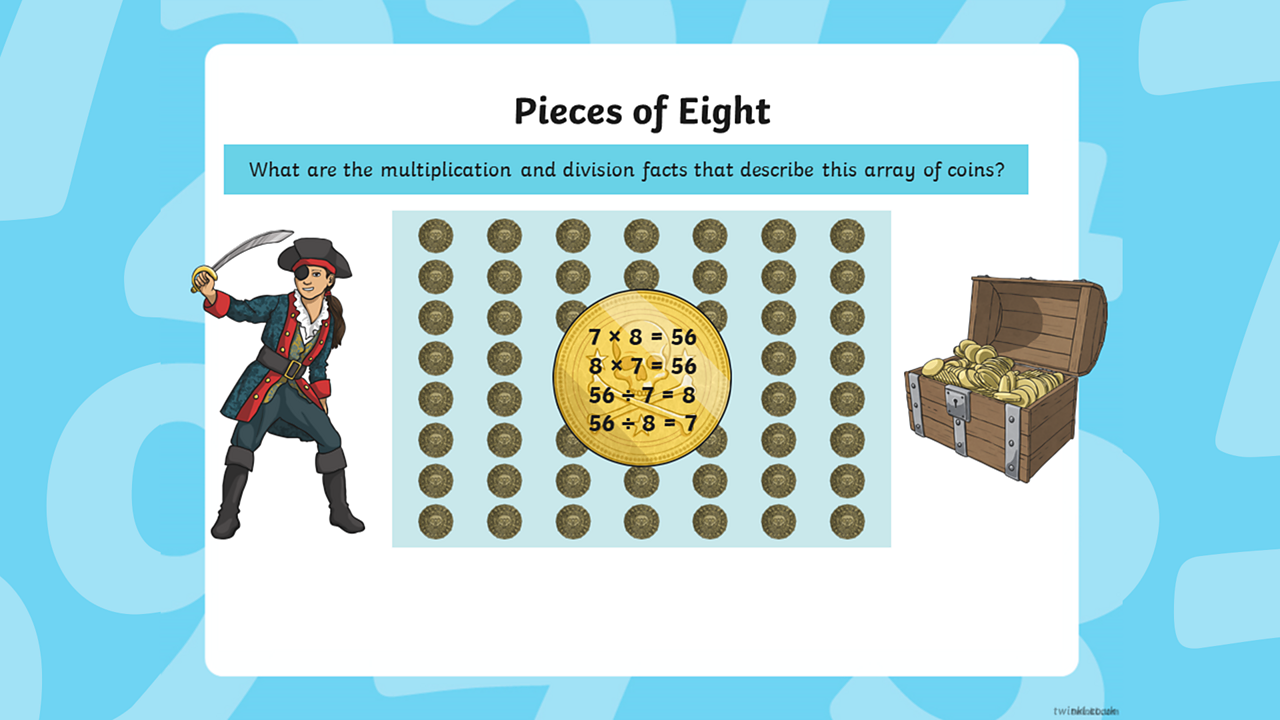The answers are 7 x 8 = 56, 8 x 7 = 56, 56 ÷ 7 = 8, 56 ÷ 8 = 79 of 9

# Practise

## Activity 1

Use these activity sheets to explore multiples of 8, multiply and divide by 8, solve simple problems and identify patterns in your 8 × table.

## Activity 2

Play this exciting space race game to help you get quicker at recalling your 8 times table.

# There's more to learn

Have a look at these other resources.